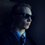# Treat $\infty$ carefully!

It is generally believed that infinity cannot be treated as a number. Otherwise, we would arrive at contradictions like this famous one:

If $\infty = \infty + 1 = \infty + 2$,

And we subtract $\infty$ from each equation,

Then $0 = 1 = 2$

However, why did we subtract only $\infty$ from each equation? If $\infty = \infty + 1 = \infty + 2$ as we first said, then couldn't we subtract $\infty$ from the first equation, $\infty + 1$ from the second, and $\infty + 2$ from the third? We wouldn't be breaking an algebraic rules, because all of these amounts are equal to each other. Then, we would get $0 = 0 = 0$ which is perfectly true!

The moral of the story: treat infinity carefully! It isn't exactly a number, but if we take certain precautions, then we can use as if it were a number.

Please note however that this again represents curious exploration, not rigid proof.

I would welcome any thoughts, comments, or objections!Note by David Stiff
1 year, 6 months ago

This discussion board is a place to discuss our Daily Challenges and the math and science related to those challenges. Explanations are more than just a solution — they should explain the steps and thinking strategies that you used to obtain the solution. Comments should further the discussion of math and science.

When posting on Brilliant:

• Use the emojis to react to an explanation, whether you're congratulating a job well done , or just really confused .
• Ask specific questions about the challenge or the steps in somebody's explanation. Well-posed questions can add a lot to the discussion, but posting "I don't understand!" doesn't help anyone.
• Try to contribute something new to the discussion, whether it is an extension, generalization or other idea related to the challenge.
• Stay on topic — we're all here to learn more about math and science, not to hear about your favorite get-rich-quick scheme or current world events.

MarkdownAppears as
*italics* or _italics_ italics
**bold** or __bold__ bold
- bulleted- list
• bulleted
• list
1. numbered2. list
1. numbered
2. list
Note: you must add a full line of space before and after lists for them to show up correctly
paragraph 1paragraph 2

paragraph 1

paragraph 2

[example link](https://brilliant.org)example link
> This is a quote
This is a quote
    # I indented these lines
# 4 spaces, and now they show
# up as a code block.

print "hello world"
# I indented these lines
# 4 spaces, and now they show
# up as a code block.

print "hello world"
MathAppears as
Remember to wrap math in $$ ... $$ or $ ... $ to ensure proper formatting.
2 \times 3 $2 \times 3$
2^{34} $2^{34}$
a_{i-1} $a_{i-1}$
\frac{2}{3} $\frac{2}{3}$
\sqrt{2} $\sqrt{2}$
\sum_{i=1}^3 $\sum_{i=1}^3$
\sin \theta $\sin \theta$
\boxed{123} $\boxed{123}$

## Comments

Sort by:

Top Newest

$∞ -∞$ is indeterminate, you can’t divide by zero, you can’t subtract ∞, you can’t divide by ∞, you can’t add ∞, can you multiply by ∞ without creating loopholes???

- 1 month ago

Log in to reply

Not sure I understand. Are you disproving, or asking a question?

- 1 month ago

Log in to reply

The first part was facts (in the sense that they create loopholes in math if defined by the normal operations), the last part was a question

- 1 month ago

Log in to reply

I see. As I mentioned, this note was mainly speculation at the time I wrote it, but since then, I've learned that there are actual formalizations of mathematics which incorporate division by zero and infinity, such as the projectively extended real line, or the more thorough wheel theory. The people who came up with them know a lot more about it then me. :)

- 4 weeks, 1 day ago

Log in to reply

×

Problem Loading...

Note Loading...

Set Loading...